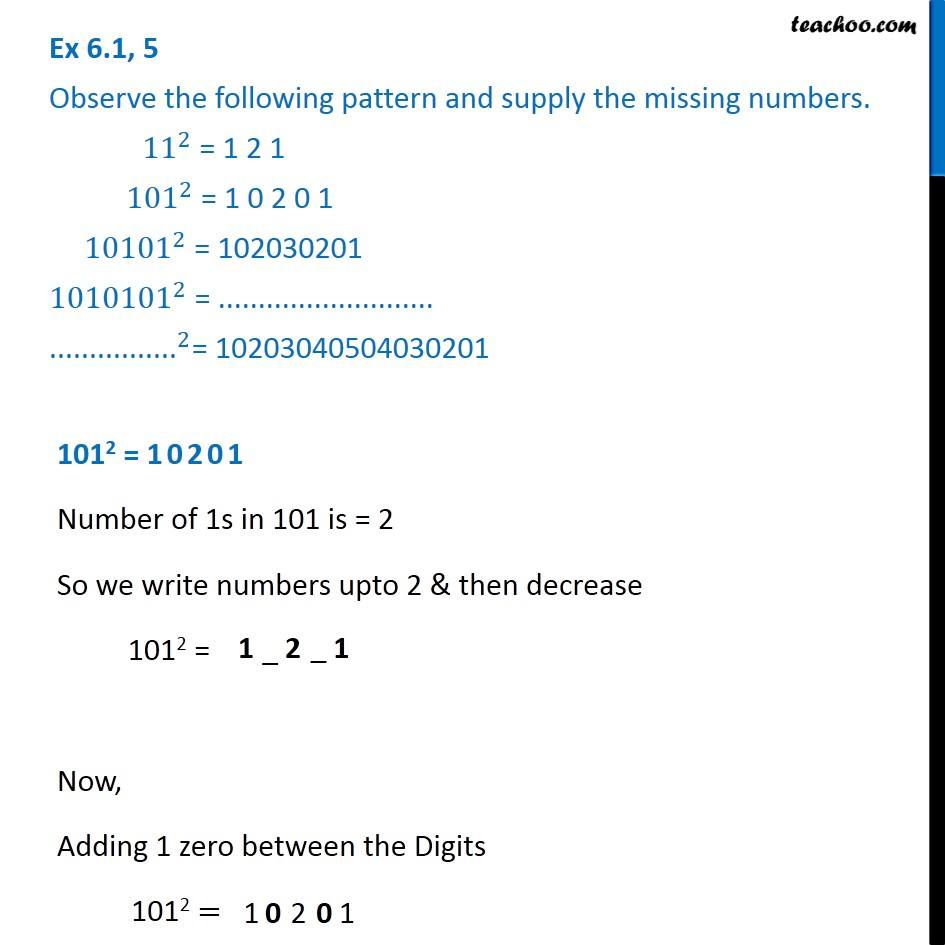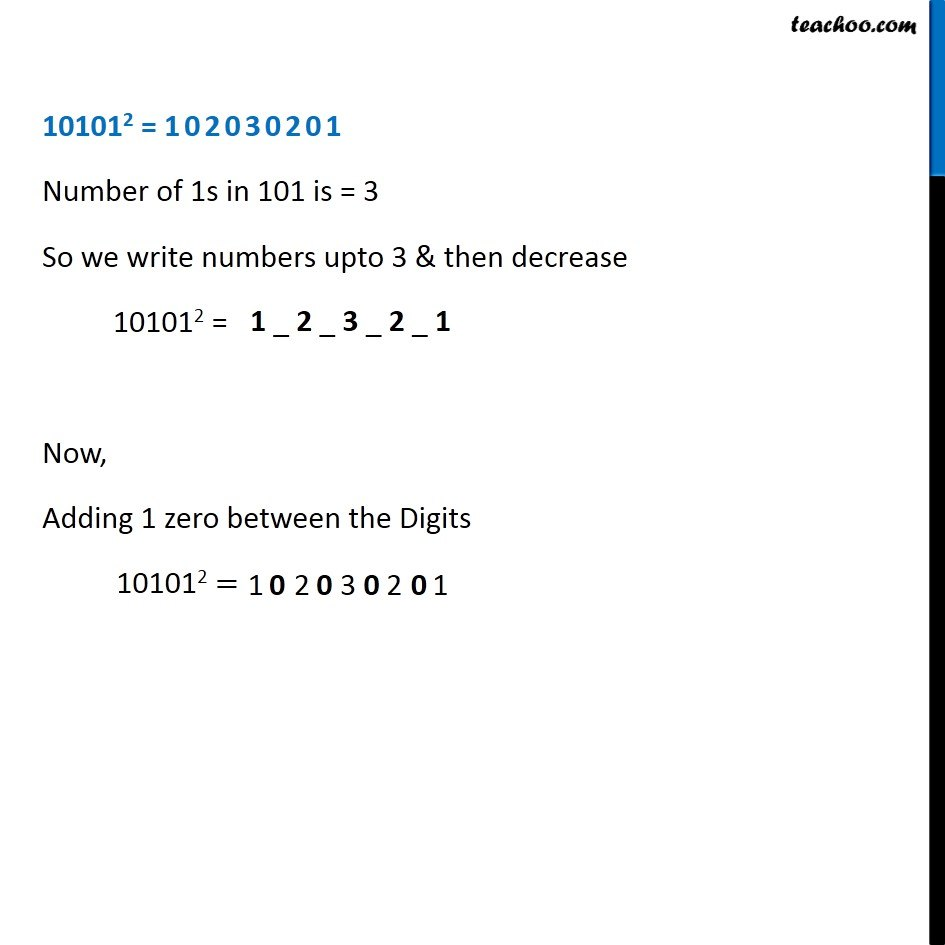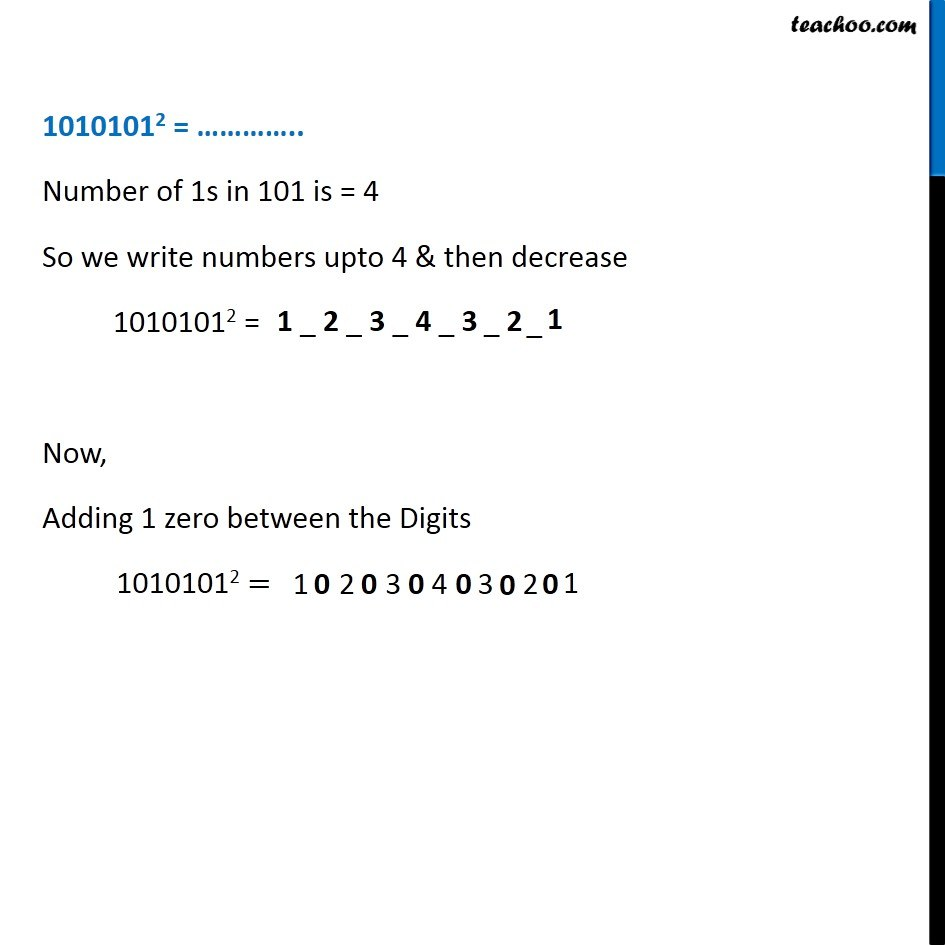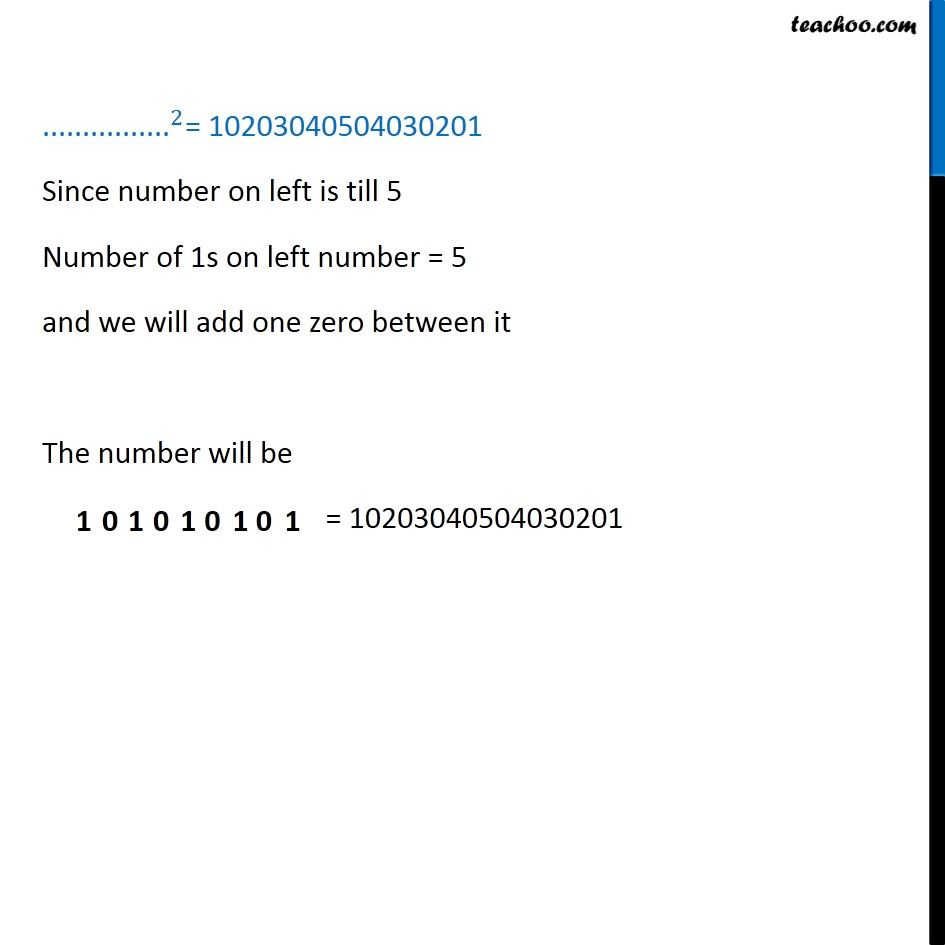Ex 5.1

Chapter 5 Class 8 Squares and Square Roots
Serial order wiseLearn in your speed, with individual attention - Teachoo Maths 1-on-1 Class

### Transcript

Ex 5.1, 5 Observe the following pattern and supply the missing numbers. 〖11〗^2 = 1 2 1 〖 101〗^2 = 1 0 2 0 1 〖 10101〗^2 = 102030201 〖1010101〗^2 = ........................... 〖"................" 〗^2= 10203040504030201 1012 = 10201 Number of 1s in 101 is = 2 So we write numbers upto 2 & then decrease 1012 = Now, Adding 1 zero between the Digits 1012 = 101012 = 102030201 Number of 1s in 101 is = 3 So we write numbers upto 3 & then decrease 101012 = Now, Adding 1 zero between the Digits 101012 = 10101012 = ………….. Number of 1s in 101 is = 4 So we write numbers upto 4 & then decrease 10101012 = Now, Adding 1 zero between the Digits 10101012 = 〖"................" 〗^2= 10203040504030201 Since number on left is till 5 Number of 1s on left number = 5 and we will add one zero between it The number will be = 10203040504030201